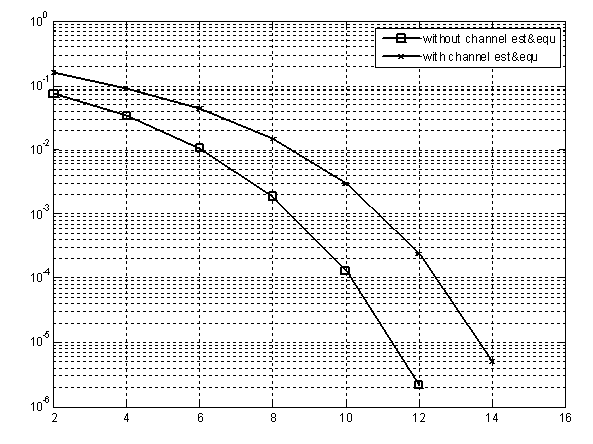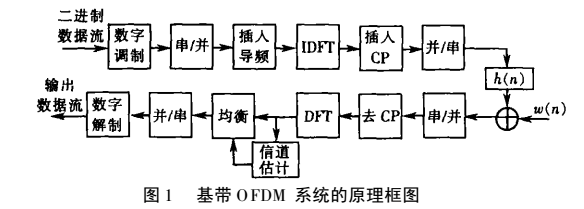•研究论文
• 进行了一下仿真，没有加信道编码，用LS信道估计和一阶均衡附件是仿真的误码率对比图，从图中可以看出均衡似乎对误码率有3dB的恶化图中横轴是SNR其中没有均衡的曲线似乎比理论曲线要好，我想这是因为我设置了一些虚拟...

在AWGN信道中没有多径
这样信道估计和均衡对结果的影响有多少dB呢？
进行了一下仿真，没有加信道编码，用LS信道估计和一阶均衡
附件是仿真的误码率对比图，从图中可以看出均衡似乎对误码率有3dB的恶化
图中横轴是SNR
其中没有均衡的曲线似乎比理论曲线要好，我想这是因为我设置了一些虚拟子载波而仿真中没有对信噪比做相应的变换吧
请教一下OFDM中信道估计和均衡处理对性能的影响有没有定量分析呢？
还有各位的仿真结果是怎么样的呢一般均衡怎么做啊
糊涂了
均衡都有哪些方式？
你怎么做信道估计和均衡的？
LS是指信道估计还是指均衡方法？
awgn信道应该不要估计了。。。
直接用awgn函数加噪声的吧
awgn信道也不存在线性卷积和循环卷积不同的问题，所以还是贴代码才好看啊
代码见附件
其中信道部分只用AWGN函数加噪声
信道估计就是把接收到的和发的导频除一下
均衡是把接收到的符号跟估计的信道除一下
AWGN信道确实不需要信道估计和均衡，所以才会有仿真结果中没有作信道估计和均衡的比有信道估计和均衡的还要好
但在接收机不能预知信道是AWGN的情况下，还是得估计和均衡啊
除的均衡效果不好
用你的方法，没有CHE的时候就是理想的CHE，由于AWGN的时候，信道响应为1，而你不用CHE的时候，就是默认CHE为1，所以理论上这两条线是重合的，所以你只要认为它是理想的就可以了，CHE还是一定要测试的，由于再AWGN无编码的时候，OFDM、CDMA、TDMA等等系统的Raw BER在等效SNR的时候应该基本一直，你可以用他们的结果做一下比较。
跟matlab的bertool产生的理想曲线对比过
没有che的结果应该是相近的
只是现在有che导致恶化3dB以上，不知道这个结果是否可靠
如果这样的话那用相关检测跟差分编码没什么区别了
现在工程应用的一般都是除的吧
相关检测和差分编码的意义区别个人觉得还是很大的，要不就是我们对这个定义的理解不太一样。
主要是我现在不好把握我这个che的仿真结果是否正常
如果大家的仿真结果也跟这个相近，那就没问题了
r u sure? hehe
你说的不做信道估计实际上就是知道信道参数为1，是已知CSI的情况，做了信道估计后的效果肯定比理想情况差吧
是啊，这里的两个结果之间其实的差距包含了信道估计的误差，均衡的误差两部分
效果肯定是会差的，请教一下这个能差多少呢？
你的图意思是理想的和实际的信道估计的差距吧？
那样的曲线是肯定是不会重合的
因为信道估计的统计量里面还有noise呢，不管是不是multipath or pure awgn
其实我现在想做的事情是仿真多径信道中的OFDM性能
但仿真结果似乎比较差，所以先确定这个仿真程序是没有问题的，就拿AWGN信道来测试，跟参考结果进行对比，发现了3dB的差别
有很多人指出过吧，你用除的方法做均衡是不好的，会放大噪声
你的意思是乘共轭？
对于硬判决来说除跟乘共轭有什么区别呢
而且我的仿真里面还没有信道编码
这个我仿真确认过了，除和乘共轭的误码率是一样的
你这个程序我跑过了，跟均衡没什么关系(qpsk乘以实的幅度因子对其误码率无影响)。简单讲来，就是因为信道估计导致的相位误差，使得qpsk星座旋转了，导致snr的衰减。
以下代码显示，选用导频为全1时，中等snr下信道估计的相位服从高斯分布：
%----------------------
clear;close all;
N = 1e6;
a = 4.0;
x = a+randn(1,N);
y = randn(1,N);
t = atan(y./x);
d = -pi/2:pi/100:pi/2;
hist(t,d);
m = mean(t);
var = mean((t-m).^2);
title(['mean = ',num2str(m),'; variance = ',num2str(var)])
%-------------------------
得到给定snr下相位的高斯分布的方差后，再找本书写相位误差下qpsk的误码率公式(matlab里就有吧)，数值积分一下，跟你的结果估计差不了。
除只是最简单的均衡吧
不管你的信道估计还是均衡，都相当于ZF方式，一般都会增加noise的效果
如果你想知道是那个模块的问题，那就这么做
理想信道估计+均衡
实际信道估计+均衡
然后和theory bound比一下就ok了
另外，OFDM的信道估计，还是MMSE的更好一些。ZF会差不少

展开全文• ## 信道估计

万次阅读 多人点赞 2018-03-08 21:59:18
前面写了关于CP在OFDM中的应用，主要是记录一点零星的想法... 2）正因为没入门，所以只能从最简单的信道估计说起，当然也会谈到CP的问题，毕竟是由于仿真CP对OFDM影响才激发了自己去看相关的材料。 3）另一个原因...
前面写了关于CP在OFDM中的应用，主要是记录一点零星的想法而已，今天突然想写点关于信道特性方面的东西。原因有以下几点：
1）信道在仿真中的地位不容置疑，不同信道的条件下的仿真是许多课题的重点，自己几乎还没入门。
2）正因为没入门，所以只能从最简单的信道估计说起，当然也会谈到CP的问题，毕竟是由于仿真CP对OFDM影响才激发了自己去看相关的材料。
3）另一个原因，写文章是整理思维的一个过程。
4）最后，Mark离我远去的智牙，疼ing!
先让我们回顾下一些小知识点：
1）信号经过多条小径到达，每条径幅度和相位随机，我们得知其幅度是服从瑞利分布，相位是均匀分布，有经典的Jakes模型以及各种改进算法来仿真（其中是考虑了多普勒频移）。
2）无线环境中的信道模型经常是多径（大径）的（直射径和反射、散射等），由于多径带来了频率选择性，所谓频率选择性，就是信道对不同频率的信号成分施加不同的影响。
3）另外，如果又外加移动的条件，那么我们的信道就是时变的了，时变就会带来频域弥散，也就是俗称的多普勒频移
4）所以，我们总会把无线信道建模为线性时变信道。

我们从最简单的多径信道开始，我们仿真有两条径，每条径并没有衰落，就是一个固定的加权值，我们先看一个例子：接下来我们用程序来验证下：

[html]
view plain
copy

<pre name="code" class="plain"><pre name="code" class="html">clear all  close all    tx_data = [1 0 0 0];  data_delay = [0 1 0 0];  rx_data = tx_data + data_delay;    fft_tx = fft(tx_data)  fft_rx = fft(rx_data)

结果如下：其实我上面的程序验证是非常非常简单的一个，如果你把数据修改为[1 2 3 4]就肯定不是这样的结果了。问题出在哪呢？这就要从公式(2)说起了，什么样的DFT才能产生那样的结果呢？回顾DSP我们可以知道，循环移位也就是周期移位才能产生公式(2)的效果,那为什么上面的程序没有循环移位又出现了正确结果呢？那是因为上面数据[1 0 0 0]线性移位和周期移位效果等同，为了验证我们来修改一下上面的数据，不妨就拿无辜的[1 2 3 4]来看看

[html]
view plain
copy

<pre name="code" class="plain"><pre name="code" class="html">clear all  close all    tx_data = [1 2 3 4];  data_delay_linear = [0 1 2 3];  data_delay_period = [4 1 2 3];  rx_data_linear = tx_data + data_delay_linear;  rx_data_period = tx_data + data_delay_period;    fft_tx = fft(tx_data)  fft_rx_linear = fft(rx_data_linear)  fft_rx_period = fft(rx_data_period)

结果如下：很明显只有循环移位叠加后的结果才满足一般的信道特性，例如k=1,(-2+2i)*H(1)=(-2+2i)*(1-i)=4i。我们在接收端只要获得了H(k)的各个值，就很容易补偿信道带来的损失了。

好了，让我们回到OFDM上来，我们把经过星座映射后的符号放置在不同的IFFT_BIN上，也就是用不同的频率来发送，在 前面博文循环前缀在OFDM中应用（一）中我们已经说明，经过信道无非是每个频率经过H(k)的加权而已，这样的话我们就不需要用那么复杂的均衡技术了，只需要估计出H(k)的值，然后逆运算就可以恢复原来的符号了，我们是用了循环前缀这一技术才能达到上面所说的效果，在那篇博文中我们是从循环前缀变线性卷积为循环卷积来证明的，但是并没有说明为什么就变换成功了，今天希望直观的理解一下。现在有了上面简单的例子，我们可以看出一点端倪。还是拿无辜的[1 2 3 4 ]来说明(在此CP长度取3)：从上面这个图，我们可以看出来只要信道冲激响应长度小于CP的长度，我们都可以把线性移位变成区间[1:4]内的循环移位，所以加了CP后的信号，经过信道传输后，我们接受端是会去掉CP那段长度的，这里还不够直观，为什么发送端加CP，接收端去掉CP就能实现循环卷积呢？好了，为了解决这个问题，让我们看看到底什么是循环卷积和线性卷积，为了直观的理解，我不打算用DSP上的理论，我从一个更加直观的方面来说。其实可以这么总结：从整体上宏观的看，我们的信号经过信道肯定是与信道的冲激响应作线性卷积的，就好比上面无辜的[1 2 3 4]例子一样，各个时延版本的加权和(这里都加权都是1而已)，但是当我们从局部微观的看，我们不只关心区间[1:4]，那么一个很显然的事实就摆正面前：在这个局部我们做的是循环卷积。
好了，我们现在的思绪差不多理清了，总结下：我们把星座映射后的符号X=[X1,X2...,Xn]经过IFFT运算后，得到了时域信号x=[x1,x2...,xn],我们加上CP后变成x'，把它变成‘宏观’的信号经过信道，整体上是一个线性卷积没错！但是在原始时域信号x那个小区间内，却始终保持的是循环卷积(只要CP长度大于信道冲激响应长度)，那么我们就可以利用手段来获取信道特征H(k),每个k就对于每个子载波上的符号Xk，所以一个频率选择性信道就变成了多个平坦的信道了。

后记：书上简单的一句：CP把线性卷积变成循环卷积，其实我认为更好的理解就是：宏观的线性卷积变成局部的循环卷积，毕竟我们接收端会去除CP，也就是说我们始终关心的还是那个局部，因为那个区间才是我们IFFT变换得来的，所以CP就是以信道带宽的代价来降低接收端的复杂度
展开全文• 对于无线通信中MIMO的信道估计与信号检测的研究。
• 该方法利用小波分解估计得到信道初始稀疏度，结合已有的压缩感知自适应算法的思想确定算法迭代停止条件完成信道估计，解决实际运用中水声通信系统信道估计时稀疏度未知的问题。仿真实验结果表明，所提出的方法可精确...压缩感知
• 本文根据双选信道在时延-多普勒域具有稀疏性,研究了OFDM系统中基于压缩感知的双选信道估计。为了克服信道的双选特性对信道估计造成的不稳定性,采用了正则正交匹配追踪(Regularized Orthogonal Matching Pursuit,ROMP...
• ## 信道估计算法

万次阅读 2016-11-10 16:59:40
信道估计算法目前我所涉及的是短波宽带无线信道下的接收端的处理，包括捕获、同步、信道估计及信道均衡，还有译码。百度百科里是这样解释这种信道的：短波通信发射电波要经电离层的反射才能到达接收设备，通信距离较...
目前我所涉及的是短波宽带无线信道下的接收端的处理，包括捕获、同步、信道估计及信道均衡，还有译码。百度百科里是这样解释这种信道的：短波通信发射电波要经电离层的反射才能到达接收设备，通信距离较远，是远程通信的主要手段。由于电离层的高度和密度容易受昼夜、季节、气候等因素的影响，所以短波通信的稳定性较差，噪声较大。因此在仿真的时候，着重仿真的是系统在加了噪声、多径、衰落情况下的接收端的性能。在接收端，捕获同步以后，信道估计就显得尤为重要，因为信道估计的好坏直接影响了后续的信道均衡性能。
对信道估计的算法也有了诸多了解，包括常见的RLS、LMS、MMSE等，还有最近在研究的压缩感知信道估计，包括贪婪算法的MP、OMP、SP以及凸优化算法中的LS0、LS0-BFGS、LS0 -FR。总的来说，对于快衰落(信道随时间变化比较快的，短波无线信道就是)信道信道的估计都需要用到已知序列。
还有另外一种系统叫多载波正交频分复用(OFDM)系统，在未知数据中插入导频（导频图案的设计也是研究的一个方向），通过频域的插值，完成信道估计。
最初，我依据Chu序列的很好的自相关性，采用了自相关法来估计信道，在信噪比高于5dB的时候，比较接近实际的信道，但是信噪比再低的时候，性能就差强人意。于是我就想有没有一种在极低信噪比情况下依然可以准确估计出信道的，于是我研究了一下压缩感知算法中的OMP算法（MP、OMP的算法理论），这种类型的压缩感知算法相较于RLS等经典的算法区别为：主要利用了信道的稀疏特性。这种算法的思想其实是将所要估计的信号通过基矩阵转换到一个能体现出其稀疏特性的域中，因为一般情况下信号是无法表现出稀疏特性的，但是，一般的信号都可以在某个域中表现出稀疏特性，这样只要此时的测量矩阵满足RIP特性就OK了。
由于时域的均衡需要计算矩阵的逆，因此复杂度比较大，因此，一般情况下，是通过加入循环前缀来形成信号与信道的循环卷积特性，从而将均衡放在频域做。循环前缀跟训练序列其实作用是不同的，一个是为了形成循环信道，频域均衡减少计算的复杂度，同时还可以作为保护间隔，避免将ISI延伸至下一个数据块。而训练序列是为了信道估计，有的人还用它做同步调整。在OFDM中，他们是两个部分，一般是先插入导频，再加入循环前缀，具体结构如下图：压缩感知信道估计主要用于OFDM系统，由于其导频是在频域体现出插值特性的，因此是做的频域信道估计。因此，可以寻求一定的基矩阵，例如傅里叶变换基，把信道从频域转化为时域，时域的信道表现出稀疏特性。从而可以用压缩感知方法恢复出来，然后再用基矩阵恢复出频域的信道。  影响压缩感知信道估计性能的因素主要有三个：  1）导频图案的选择。导频图案的选择和长度决定了观测矩阵的导频图案的选择，决定了压缩感知算法中观测矩阵的形状。  2）导频图案的长度。由于压缩感知技术实际上是一种数据压缩技术，而当把压缩感知技术应用到信道估计中时，稀疏多径信道就变成了原始数据，导频信息经过信道的过程就变成了数据观测的过程，接收到的导频信息就变成了压缩后的数据，而数据的长度 N相当于信道的长度，压缩后的数据长度 M 相当于导频数量，对于稀疏多径信道来说，显然N>>M。因此，使用压缩感知技术的信道估计，只需较少的导频数量，就能得到信道的完整估计。  3）信号重构算法。使用不同的重构算法，都会使信道估计的性能有所差异。
而在SC系统中，用训练序列直接就可以在时域估计信道，而且由于循环前缀的存在，观测矩阵为拓普利兹矩阵，也满足RIP特性，因此可以用压缩感知方法，就不需要转换域了。仅仅依靠信号重构算法的不同带来的好处有多少，我本人也不是非常清楚。但是根据我对压缩感知算法中的OMP、SP、LS0、LS0-FR、LS0-BFGS算法仿真结果来看，性能跟自相关法是一样的。仿真得到的信道就是发送的训练序列的循环矩阵的逆与接收到的训练序列的乘积。  到现在为止，我对一点弄不明白，就是时域均衡的话，对于训练序列而言，接收到的训练序列跟发送的训练序列都是已知的话，那么不论用哪种方法，所估计出来的信道都大体是一样的，这样估计出来的信道包括了噪声带来的误差等，如何能在极低的信噪比情况下估计出最逼近真实信道的信道，到目前为止，我还不清楚，能不能做到，我也不清楚。这是到目前为止我对信道估计的一点认识和疑惑。如有问题敬请指出。
展开全文• 瑞利信道基带信号均衡仿真，绘制QPSK和16QAM理论误码率和误符号率
• 针对采用非正交多载波调制的无线通信系统中，信道的稀疏度未知且非零稀疏路径随时间变化的问题，提出了一种基于导频信号互相关运算的压缩感知信道估计方法，利用接收端导频信号互相关计算对信道 稀疏度及非零稀疏...
• 针对煤矿井下信道情况复杂,多径效应会带来噪声增加和误码率上升等问题,建立了煤矿井下正交频分复用系统模型,研究了井下正交频分复用信道最小平方、最小均方误差估计算法;在矩形导频分配方案的基础上,提出了在时域...
• 理论分析与计算机仿真表明，该方法能够有效地降低加性噪声对信道估计的影响，提高信道估计精度。在采用QPSK调制的一发四收的接收分集OFDM系统中，该增强型的信道估计方法的性能明显好于最小二乘信道估计和基于DFT的...
• 效的二维OFDM信道估计算法。首先，本文对两种基于FFT的多导频信道估计算 法进行了深入的研究，针对移动信道的特点对其进行了改进，进一步降低了系统 误码率。然后根据EIDCT理论，设计出一种新的插值算法，并将其应用...
• 针对频率选择性衰落信道下的放大转发协同正交频率复用（OFDM）通信系统，提出一种基于压缩感知理论的稀疏信道估计方法。首先，构造协同OFDM系统模型，利用循环矩阵理论，将该系统模型变换成类似于传统的点对点系统...
• 这个文件里面包含了5篇paper，都是关于信道均衡和误码率方面的paper，是国外著名的trans上的paper,质量高，非常值得研究
• 参考： 请参见Simon Haykin所著的“自适应滤波器理论”一书中的第9章matlab
• 单载波频域均衡系统因具有峰均比低、适用于高速率传输的优势，具有广泛...理论分析及仿真结果表明，本文算法比传统的LS及MMSE信道估计算法在未编码系统及编码系统中的性能都更好，且运算复杂度比传统一维MMSE算法更低。单载波频域均衡 SVD 特殊字
• 为了研究双选择性瑞利信道中信道估计误差对MIMO-OFDM系统的影响,使用迫零检测和对Wishart分布的近似处理,从理论上推导出了存在信道估计误差时双选择不相关瑞利信道和双选择指数相关瑞利信道中MIMO-OFDM系统信干噪比...
• 人工智能在移动通信网络中的应用：基于机器学习理论信道估计与信号检测算法.pdf
• 大规模MIMO信道估计


文章目录
5.1 大规模MIMO信道估计与导频设计基本原理5.1.1 信道估计原理5.1.2 导频设计基本原理
5.2 传统信道估计方法5.2.1 LS信道估计5.2.2 LMMSE信道估计
5.3 低复杂度的大规模MIMO信道估计参考文献

本文内容摘录自《大规模MIMO传输理论与关键技术》与《MIMO-OFDM无线通信技术及MATLAB实现》

5.1 大规模MIMO信道估计与导频设计基本原理
信道状态信息(CSI) 是一种笼统的概念，它包括信道矩阵。只要是反应Channel的都叫信道状态信息。信道矩阵只是MIMO系统中百的一种信道状态信息。其他的比如Channel profile，多径时延，多普勒频偏，MIMO信道的秩，波束形成向量等等，都属于信道状态信息。当前的信道矩阵H只能算是一种信道状态信息，但是是最常用的。
在大规模MIMO中，接收端的信道均衡和符号检测都需要精确的CSI。并且，只有在准确知道传播环境的情况下才可以实现空间的高分辨率。因此，准确的信道估计成为限制大规模MIMO系统性能的一个主要因素。信道估计是指接收端根据一定的准则，从接收数据中将某个信道模型的模型参数估计出来的过程。传统的基于导频的信道估计方法有最小二乘法（Least Square，LS）、MMSE、线性最小均方误差（Linear Minimum Mean Square Error，LMMSE）法和极小方差无偏（Minimum Variance Unbiased，MVU）估计等，这些方法一般都包括矩阵求逆的过程。在大规模MIMO中，由于信号的维度较大，传统的信道估计方法计算复杂度过高，因此需要采用复杂度较低的信道估计方法。
5.1.1 信道估计原理
在TDD模式下，上行通信和下行通信采用相同的频段，并假设上行信道和下行信道具有互易性。以一个小区为例，小区内的各个用户采用同步方式通过上行链路发送导频序列，小区内的基站接收这些导频序列并进行相应的处理得到上行信道的信道估计，然后再由下行链路传输信道信息与数据信息

[

1

]

^{}

。FDD模式下上行通信和下行通信分别采用不同的频段，因此上行信道与下行信道不具有互易性。由于FDD模式是3G通信系统和4G通信系统中的主流模式，如果在FDD模式下运用大规模MIMO，将有利于大规模MIMO在5G通信系统中的推广。5.1.2 导频设计基本原理
理论研究发现，当基站（Base Station，BS）的天线数无限增加时，热噪声和小区内其他用户干扰对信道估计和符号检测的影响均会消失，限制性能的唯一因素是在其他小区复用了期望小区的导频序列而引起的同频干扰，这一现象称为导频污染（Pilot Contamination，PC）

[

2

]

^{}

。
当前用于大规模MIMO技术中的导频设计为

[

3

,

4

]

^{[3,4]}

：小区内采用正交导频，相邻小区采用非正交导频（即导频复用）。该导频设计方法使得同一小区内各个用户的导频序列正交，消除了多用户干扰；而同一组导频序列可被不同小区采用，从而提高频谱效率。
由于FDD模式下进行信道估计需要占用较多的频谱资源，目前针对大规模MIMO的信道估计研究主要集中在TDD模式下。当天线数量无限增大时，导频污染问题成为制约系统性能的唯一因素。如何解决导频污染问题亦成为导频设计与信道估计的关键。
5.2 传统信道估计方法
训练符号可以用于信道估计，通常能够提供较好的性能。然而，除了发射数据符号外， 还需要发射前导或导频信号，由此产生的负荷会降低传输效率.当可以获得训练符号时，最小二乘( LS )和线性最小均方误差(LMMSE) 技术被广泛应用于信道估计。
5.2.1 LS信道估计
假设所有子载波是正交的，即没有ICl，那么可以将N 个子载波的训练符号表示成矩阵形式：

X

=

[

X

[

0

]

0

⋯

0

0

X

[

1

]

⋮

⋮

⋱

0

0

⋯

0

X

[

N

−

1

]

]

\mathbf{X}=\left[\begin{array}{cccc} X & 0 & \cdots & 0 \\ 0 & X & & \vdots \\ \vdots & & \ddots & 0 \\ 0 & \cdots & 0 & X[N-1] \end{array}\right]

其中

X

[

k

]

X[k]

表示第

k

k

个子载波上的导频信号，满足

E

{

X

[

k

]

}

=

0

,

Var

⁡

{

X

[

k

]

}

=

σ

2

,

k

=

0

,

1

,

2

,

⋯

,

N

−

1

,

E\{X[k]\}=0,\operatorname{Var}\{X[k]\}=\sigma^{2},k=0,1,2, \cdots, N-1,

因为假设所有的子载波都是正交的，所以

X

\mathbf X

是一个对角矩阵。给定策

k

k

个载波的信道增益

H

[

k

]

H[k]

，接收到的训练信号

Y

[

k

]

Y[k]

能的表示为

Y

≜

[

Y

[

0

]

Y

[

1

]

⋮

Y

[

N

−

1

]

]

=

[

X

[

0

]

0

⋯

0

0

X

[

1

]

⋮

⋮

⋱

0

0

⋯

0

X

[

N

−

1

]

]

[

H

[

0

]

H

[

1

]

⋮

H

[

N

−

1

]

]

+

[

Z

[

0

]

Z

[

1

]

⋮

Z

[

N

−

1

]

]

=

X

H

+

Z

\begin{array}{rl} \mathbf{Y} & \triangleq \left[\begin{array}{c} Y \\ Y \\ \vdots \\ Y[N-1] \end{array}\right] \\ \end{array}= \left[\begin{array}{cccc} X & 0 & \cdots & 0 \\ 0 & X & & \vdots \\ \vdots & & \ddots & 0 \\ 0 & \cdots & 0 & X[N-1] \end{array}\right]\left[\begin{array}{c} H \\ H \\ \vdots \\ H[N-1] \end{array}\right]+\left[\begin{array}{c} Z \\ Z \\ \vdots \\ Z[N-1] \end{array}\right] =\mathbf{X} \mathbf{H}+\mathbf{Z}

其中，信道向量

H

=

[

H

[

0

]

,

H

[

1

]

,

⋯

,

H

[

N

−

1

]

]

T

\mathbf{H}=[H, H, \cdots, H[N-1]]^{T}

；噪声向量

Z

=

[

Z

[

0

]

,

Z

[

1

]

,

⋯

Z

[

N

−

1

]

]

T

,

\mathbf{Z}=[{Z}, Z, \cdots Z[N-1]]^{T},

满足

E

{

Z

[

k

]

}

=

0

,

Var

⁡

{

Z

[

k

]

}

=

σ

z

2

,

k

=

0

,

1

,

2

,

⋯

,

N

−

1

E\{Z[k]\}=0, \operatorname{Var}\{Z[k]\}=\sigma_{z}^{2},k=0,1,2, \cdots, N-1

，在接下来的讨论中， 令

H

^

\hat{\mathbf H}

表示对信道

H

\mathbf {H}

的估计。
假设所有子载波是正交的，即没有ICI。

ICI：多载波通信系统对载波频率偏移十分敏感，尤其对于多用户并行传输的无线通信上行链路，不同用户的发射信号具有不同的频率偏移，这种由多个独立频偏导致的子载波间干扰（Inter-Carrier Interference，ICI）问题较为严重，必须设计出高效的信号处理方法加以抑制。此外，在高速移动的无线信道下，由于多普勒扩展很大，信道将在一个多载波符号内产生时变，由此引起的 ICI 问题也将十分严重。

为了得到信道估计

H

^

\hat {\mathbf H}

，LS 信道估计法需要最小化下面的代价的数：

J

(

H

^

)

=

∥

Y

−

X

H

^

∥

2

=

t

r

{

(

Y

−

X

H

^

)

H

(

Y

−

X

H

^

)

}

=

t

r

{

Y

H

Y

−

Y

H

X

H

^

−

H

^

H

X

H

Y

+

H

^

H

X

H

X

H

^

}

\begin{aligned} J(\hat{\mathbf{H}}) &=\|\mathbf{Y}-\mathbf{X} \hat{\mathbf{H}}\|^{2} \\ &=tr\{(\mathbf{Y}-\mathbf{X} \hat{\mathbf{H}})^{{H}}(\mathbf{Y}-\mathbf{X} \hat{\mathbf{H}}) \}\\ &=tr\{\mathbf{Y}^{{H}} \mathbf{Y}-\mathbf{Y}^{{H}} \mathbf{X} \hat{\mathbf{H}}-\hat{\mathbf{H}}^{{H}} \mathbf{X}^{{H}} \mathbf{Y}+\hat{\mathbf{H}}^{{H}} \mathbf{X}^{{H}} \mathbf{X} \hat{\mathbf{H}}\} \end{aligned}

令上面的代价函数关于

H

^

\hat{\mathbf H}

的偏导数等于 0，即

∂

J

(

H

^

)

∂

H

^

=

−

2

(

X

H

Y

)

+

2

(

X

H

X

H

^

)

=

0

\frac{\partial J(\hat{\mathbf{H}})}{\partial \hat{\mathbf{H}}}=-2\left(\mathbf{X}^{{H}} \mathbf{Y}\right)+2\left(\mathbf{X}^{{H}} \mathbf{X} \hat{\mathbf{H}}\right)=0

然后可以得到

X

H

X

H

^

=

X

H

Y

\mathbf X^H\mathbf X\hat{\mathbf H}=\mathbf{X}^{{H}} \mathbf{Y}

，由此得到 LS 信道估计的解为

H

^

L

S

=

(

X

H

X

)

−

1

X

H

Y

=

X

−

1

Y

\hat{\mathbf{H}}_{{LS}}=\left(\mathbf{X}^{{H}} \mathbf{X}\right)^{-1} \mathbf{X}^{{H}} \mathbf{Y}=\mathbf{X}^{-1} \mathbf{Y}

注意上式中，

X

−

1

\mathbf{X}^{-1}

表示导频信号矩阵

X

\mathbf{X}

的逆或伪逆。
令

H

^

L

S

[

k

]

\hat{H}_{{LS}}[k]

表示

H

^

L

S

\hat{\mathbf{H}}_{{LS}}

中的元素，

k

=

0

,

1

,

2

,

⋯

,

N

−

1

k=0,1,2, \cdots, N-1

，由无ICI 的假设条件可知

X

\mathbf X

为对角矩阵，因此每个子载波上的 LS信道估计可以表示为

H

^

L

S

[

k

]

=

Y

[

k

]

X

[

k

]

,

k

=

0

,

1

,

2

,

⋯

,

N

−

1

LS 信道估计的均方误差(MSE) 为

MSE

⁡

L

S

=

E

{

t

r

{

(

H

−

H

^

L

S

)

H

(

H

−

H

L

S

)

}

}

=

E

{

t

r

{

(

H

−

X

−

1

Y

)

H

(

H

−

X

−

1

Y

)

}

}

=

E

{

t

r

{

(

X

−

1

Z

)

H

(

X

−

1

Z

)

}

}

=

E

{

t

r

{

Z

H

(

X

X

H

)

−

1

Z

}

}

=

σ

z

2

σ

x

2

\begin{aligned} \operatorname{MSE}_{{LS}} &=E\left\{tr\left\{ \left(\mathbf{H}-\hat{\mathbf{H}}_{{LS}}\right)^{{H}}\left(\mathbf{H}-\mathbf{H}_{{LS}}\right)\right\} \right\}\\ &=E\left\{tr\left\{ \left(\mathbf{H}-\mathbf{X}^{-1} \mathbf{Y}\right)^{{H}}\left(\mathbf{H}-\mathbf{X}^{-1} \mathbf{Y}\right)\right\} \right\} \\ &=E\left\{tr\left\{\left(\mathbf{X}^{-1} \mathbf{Z}\right)^{H}\left(\mathbf{X}^{-1} \mathbf{Z}\right)\right\} \right\}\\ &=E\left\{tr\left\{\mathbf{Z}^{{H}}\left(\mathbf{X X}^{{H}}\right)^{-1} \mathbf{Z}\right\} \right\} \\ &=\frac{\sigma_{z}^{2}}{\sigma_{x}^{2}} \end{aligned}

注意，上式中的MSE 与信噪比

σ

x

2

/

σ

z

2

{\sigma_{x}^{2}}/{\sigma_{z}^{2}}

成反比， 这意味着LS 估计增强了噪声，在信道处于深度衰落时更是如此。然而， LS 方法由于简单而被广泛应用于信道估计。
5.2.2 LMMSE信道估计
考虑5.2.1中的LS 解，即

H

^

L

S

=

X

−

1

Y

≜

H

~

\hat{\mathbf H}_{{LS}}=\mathbf X^{-1} \mathbf Y \triangleq \tilde{\mathbf H}

，利用加权矩阵

W

\mathbf W

，定义LMMSE估计为

H

^

≜

W

H

~

\hat{\mathbf H} \triangleq \mathbf W \tilde{\mathbf H}

。根据图6.4 ，LMMSE 信道估计

H

^

\hat{\mathbf H}

的MSE可以表示为

J

(

H

^

)

=

E

{

∥

e

∥

2

}

=

E

{

∥

H

−

H

^

∥

2

}

J(\hat{\mathbf H})=E\left\{\|\mathbf e\|^{2}\right\}=E\left\{\|\mathbf H-\hat{\mathbf H}\|^{2}\right\}在LMMSE信道估计中， 通过选择

W

\mathbf W

最小化上式中的MSE ，可以证明估计误差向量

e

=

H

−

H

^

\mathbf e=\mathbf{H}-\hat{\mathbf{H}}

与

H

~

\tilde{\mathbf {H}}

正交，即满足

E

{

e

H

~

H

}

=

E

{

(

H

−

H

^

)

H

~

H

}

=

E

{

(

H

−

W

H

~

)

H

~

H

}

=

E

{

H

H

~

H

}

−

W

E

{

H

~

H

~

H

}

=

R

H

H

~

−

W

R

H

~

H

~

=

0

\begin{aligned} E\left\{\mathbf e \tilde{\mathbf{H}}^{H}\right\} &=E\left\{(\mathbf{H}-\hat{\mathbf{H}}) \tilde{\mathbf{H}}^{\mathrm{H}}\right\} \\ &=E\left\{(\mathbf{H}-\mathbf{W} \tilde{\mathbf{H}}) \tilde{\mathbf{H}}^{\mathrm{H}}\right\} \\ &=E\left\{\mathbf{H} \tilde{\mathbf{H}}^{\mathrm{H}}\right\}-\mathbf{W} E\left\{\tilde{\mathbf{H}} \tilde{\mathbf{H}}^{\mathrm{H}}\right\} \\ &=\mathbf {R}_{\mathbf{H} \tilde{\mathbf{H}}}-\mathbf W \mathbf R_{\tilde{\mathbf{H}} \tilde{\mathbf H}}=\mathbf {0} \end{aligned}

其中，

R

A

B

\mathbf{R}_{A B}

为矩阵

A

\mathbf{A}

和

B

\mathbf{B}

的互相关矩阵，即

R

A

B

=

E

{

A

B

H

}

\mathbf{R}_{\mathbf A \mathbf B}=E\left\{\mathbf A \mathbf{B}^{{H}}\right\}

，

H

~

\tilde {\mathbf{H}}

为LS信道估计：

H

~

=

X

−

1

Y

=

H

+

X

−

1

Z

\tilde{\mathbf{H}}=\mathbf{X}^{-1} \mathbf{Y}=\mathbf{H}+\mathbf{X}^{-1} \mathbf{Z}

于是可得，

W

=

R

H

H

~

R

H

~

H

~

−

1

\mathbf{W}=\mathbf{R}_{\mathbf{H} \tilde{\mathbf{H}}} \mathbf{R}_{\tilde{\mathbf{H}} \tilde{\mathbf{H}}}^{-1}

其中，

R

H

~

H

~

\mathbf{R}_{\tilde{\mathbf{H}} \tilde{\mathbf{H}}}

为

H

~

\tilde{\mathbf {H}}

的自相关矩阵，即

R

H

~

H

~

=

E

{

H

~

H

~

H

}

=

E

{

X

−

1

Y

(

X

−

1

Y

)

H

}

=

E

{

(

H

+

X

−

1

Z

)

(

H

+

X

−

1

Z

)

H

}

=

E

{

H

H

H

+

X

−

1

Z

H

H

+

H

Z

H

(

X

−

1

)

H

+

X

−

1

Z

Z

H

(

X

−

1

)

H

}

=

E

{

H

H

H

}

+

E

{

X

−

1

Z

Z

H

(

X

−

1

)

H

}

=

E

{

H

H

H

}

+

σ

z

2

σ

x

2

I

\begin{aligned} \mathbf{R}_{\tilde{\mathbf H} \tilde{\mathbf H}} &=E\left\{\tilde{\mathbf{H}} \tilde{\mathbf{H}}^{\mathrm{H}}\right\} \\ &=E\left\{\mathbf{X}^{-1} \mathbf{Y}\left(\mathbf{X}^{-1} \mathbf{Y}\right)^{\mathrm{H}}\right\} \\ &=E\left\{\left(\mathbf{H}+\mathbf{X}^{-1} \mathbf{Z}\right)\left(\mathbf{H}+\mathbf{X}^{-1} \mathbf{Z}\right)^{\mathrm{H}}\right\} \\ &=E\left\{\mathbf{H H}^{\mathrm{H}}+\mathbf{X}^{-1} \mathbf{Z} \mathbf{H}^{\mathrm{H}}+\mathbf{H} \mathbf{Z}^{\mathrm{H}}\left(\mathbf{X}^{-1}\right)^{\mathrm{H}}+\mathbf{X}^{-1} \mathbf{Z} \mathbf{Z}^{\mathrm{H}}\left(\mathbf{X}^{-1}\right)^{\mathrm{H}}\right\} \\ &=E\left\{\mathbf{H H}^{\mathrm{H}}\right\}+E\left\{\mathbf{X}^{-1} \mathbf{Z Z}^{\mathrm{H}}\left(\mathbf{X}^{-1}\right)^{\mathrm{H}}\right\} \\ &=E\left\{\mathbf{H H}^{\mathrm{H}}\right\}+\frac{\sigma_{z}^{2}}{\sigma_{x}^{2}} \mathbf{I} \end{aligned}

R

H

H

~

\mathbf{R}_{\mathbf{H} \tilde{\mathbf{H}}}

是频域上真实信道向量和临时信道估计向量之间的互相关矩阵，根据上式，LMMSE信道估计可以表示为

H

^

L

M

M

S

E

=

W

H

~

=

R

H

H

~

R

H

~

H

~

−

1

H

~

=

R

H

H

~

(

R

H

H

+

σ

z

2

σ

x

2

I

)

−

1

H

~

\begin{aligned} &\hat{\mathbf{H}}_{LMMSE}=\mathbf{W} \tilde{\mathbf{H}}=\mathbf{R}_{\mathbf{H} \tilde{\mathbf{H}}} \mathbf{R}_{\tilde{\mathbf{H}} \tilde{\mathbf H}}^{-1} \tilde{\mathbf{H}}\\ &=\mathbf{R}_{\mathbf{H} \tilde{\mathbf{H}}}\left(\mathbf{R}_{\mathbf{H} {\mathbf{H}}}+\frac{\sigma_{z}^{2}}{\sigma_{x}^{2}}\mathbf I\right)^{-1} \tilde{\mathbf H} \end{aligned}

5.3 低复杂度的大规模MIMO信道估计
基于导频的信道估计算法如LS算法、MMSE算法、LMMSE算法和MVU算法等都包括矩阵求逆的过程。如果发射天线与接收天线均不存在相干性，则信道的协方差矩阵为对角矩阵，矩阵求逆的复杂度较低。然而，由于大规模MIMO天线数量大，天线之间的间隔距离难以满足半波长要求，加上复杂的电波传播环境，使得天线之间具有明显的相关性，因此信道的协方差矩阵不能近似为对角矩阵。基于以上原因，大规模MIMO的信道估计中，矩阵求逆的计算复杂度非常大，因此传统的信道估计方法不能直接应用在大规模MIMO中，必须采用低复杂度的大规模MIMO信道估计方法。
参考文献
 Ngo H Q, Larsson E G, Marzetta T L. Energy and spectral efficiency of very large multiuser MIMO systems[J]. Communications, IEEE Transactions on, 2013, 61(4): 1436-1449.  Jose J, Ashikhmin A, Marzetta T L, et al. Pilot contamination and precoding in multi-cell TDD systems[J]. Wireless Communications, IEEE Transactions on, 2011, 10(8): 2640-2651.  H. Q. Ngo, M. Matthaiou, T. Q. Duong, E. G. Larsson. ‘‘Uplink performance analysis of multicellmu- mimo systems with zf receivers.” IEEE Trans. Veh. Technol., vol. 62, no. 9, Nov. 2013.  H. Q. Ngo, E. G. Larsson, T. L. Marzetta. ‘‘The Multicell Multiuser MIMO Uplink with Very Large Antenna Arrays and a Finite-Dimensional Channel.” Communications, IEEE Trans. Commun., vol. 61, no.6, pp.2350-2361, June 2013.

博主不定期发布『保研/推免、C/C++、5G移动通信、Linux、生活随笔』系列文章，如果觉得本文对你有帮助，可以『点赞＋关注』支持一下哦！

展开全文大规模MIMO LS算法 LMMSE算法 5G关键技术
• 为了提高超宽带接收机性能,提出一种适用于TH-PPM调制方式的超宽带信道估计算法,并对其误码率...通过对该算法性能的理论分析及计算机仿真实验表明，该算法在保证信道估计性能的前提下具有低复杂度,易于工程实现的优点。递归最小二乘
• 摘 要：针对基于扩展模型(BEM)的正交频分复用(OFDM)系统时变信道估计中频谱利用率不高的问题，提出了一种多符号BEM方法。相对于传统的单符号BEM方法，提出的方法减少了导频的子载波，并且通过基线倾斜技术抑制了...正交频分复用 基扩展模型
• 针对上述问题，提出一种结合分布式压缩感知理论信道估计导频优化方案。首先，根据时延域中无线信道的稀疏特性挖掘基函数系数之间的联合稀疏性并对估计方程进行去耦处理。接着，引入分布式压缩感知理论，获得一种...分布式压缩感知
• 针对LTER通信系统，对快时变信道估计问题进行了研究。采用基扩展模型对高速铁路通信环境的快时变信道进行拟合，将信道冲激响应建模为基函数与系数相乘形式。通过理论推导，得到最优基函数系数。仿真结果表明，泛化...
• 采用基于信号处理领域最近兴起的压缩感知理论对NC-OFDM系统进行信道估计，并将回溯迭代自适应正交匹配追踪算法（BAOMP）应用到NC-OFDM系统的信道估计中，该算法在每次回溯迭代中核查所选原子的可靠性并删除不可靠...
• 根据FMT系统的特点，研究了在FMT系统中进行信道估计的方法。...理论分析和计算机仿真结果均表明，在时域中对FMT系统进行信道估计简单有效，并且基于扩额序列估计的性能优于LS估计，改进后的估计方法进一步提高了性能。
• 参考： 参见 Simon Haykin 所著“自适应滤波器理论”一书中的第 8 章matlab
• 针对快时变信道估计参数多且估计复杂度大的问题，提出了一种基于高次数据拟合的快时变信道估计算法。该方法首先利用导频的周期性，在时域对一个OFDM符号内的信道进行分段估计，以减少时变信道被估参数；并且基于高次...正交频分复用 时变信道
• compressive sensing)理论的不连续子载波正交频分复用(NC-OFDM,non-contiguous orthogonal frequency division multiplexing)系统信道估计新方法,全面研究了认知无线电NC-OFDM系统CS信道估计理论框架、导频图案的......